# Batch Normalization¶

The Batch Normalization technique (https://arxiv.org/abs/1502.03167) is a commonly used method to accelerate training of deep networks. It is thought to reduce "covariate shift", or the tendency for different activations to be scaled arbitrarily which makes using a fixed learning rate for all activations difficult. The idea is based on the standard whitening trainform but it also includes two learnable parameters which allow the layers to still learn complex transformations of the input.

Statistics are computed over the mini-batch, for an activation of size $n \times w \times h \times c$ for $n$ samples in the batch, $w \times h$ activations, and $c$ channels, the transformation treats this is $n \cdot w \cdot h$ samples each of dimension $c$.

The following is standard boilerplate code and can be ignored.

In :
!pip install tabulate

import numpy as np
import matplotlib.pyplot as plt
from IPython.display import display, HTML
from tabulate import tabulate

def show_image(m, ax=None):
c_img = np.zeros((m.shape, m.shape, 3))

max_gr0 = np.max(m[m > 0])

if len(m[m < 0]) > 0:
min_le0 = np.min(m[m < 0])
else:
min_le0 = 0

c_img[m < 0] = np.array([[0.0, 1.0, 0.0]]) * (m[m < 0] / min_le0).reshape(-1, 1)
c_img[m == 0] = np.array([0.0, 0.0, 1.0])
c_img[m > 0] = np.array([[1.0, 0.0, 0.0]]) * (m[m > 0] / max_gr0).reshape(-1, 1)

plt.grid(False)

if ax is None:
return plt.imshow(c_img)
else:
ax.grid(False)
return ax.imshow(c_img)

def show_batch(batch):
plt.figure(figsize=(15, 10))
for b in range(batch.shape):
for c in range(batch.shape):
plt.subplot(batch.shape, batch.shape, b * batch.shape + c + 1)
show_image(batch[b, c, :, :])

def show_mat(m):
latex = tabulate(m, tablefmt="html",floatfmt=".3f")
display(HTML(data=latex))

Requirement already satisfied: tabulate in /usr/local/lib/python3.6/dist-packages (0.8.2)


## Batch Data¶

We start by generating a batch of "images" to demonstrate the algorithm on. The images are random $8 \times 8$ images in the range $[0, 255)$. For simplicities sake, three channels per batch ($c=3$) are used and the batch size is 2 ($n=2$). The batch is displayed using the standard convention of red pixels being positive, blue pixels being 0, and green pixels being negative. Note that there are no green pixels in the original batch. Samples are displayed on each row, so the first row shows the three channels in the first sample, the second row shows the three channels in the second sample.

In :
def generate_batch(n, c):
return np.random.randint(0, 255, size=(n, c, 8, 8)).astype(float)

batch = generate_batch(4, 3)
show_batch(batch)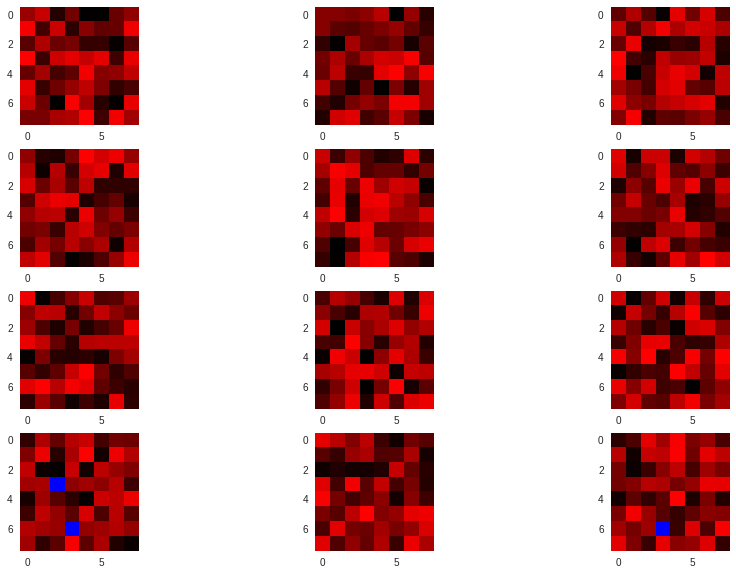## Spatial Domain Batch Normalization¶

Now the original batch normalization algorithm is implemented. This is an extremely simple algorithm. Given the samples $x$ the learnable parameters $\gamma$ and $beta$, the batch normalization $y$ of $x$ is computed by:

1. Take the mean of the samples $\mu$
2. Take the std deviation of the samples $\sigma$
3. Subtract the mean from each image and divide by the std deviation: $\hat{x} = \frac{x - \mu}{\sigma}$
4. Compute the final scale and shift using the learnable parameters: $y = \gamma \hat{x} + \beta$.

This is implemented below. The original mean and std. dev are printed as well as the mean and std dev after applying the transform. Note that the $\gamma$ and $\beta$ parameters are left off for this demonstration ($\gamma = 1$, $\beta = 0$) as their specific values are not important right now. We will revisit them later. The visual result now shows green pixels which demonstrates the re-centering of the data around a 0 mean. Note that when computing the variance, we use a blockwise formulation and then combine the block variances to obtain the population variance. This is not strictly necessary for the spatial domain formulation but it is for the DCT formulation as will be explained later.

In :
def batch_norm(batch):
batch_channels = batch.transpose((1, 0, 2, 3))

m = np.mean(batch_channels, axis=(1, 2, 3))

m_blocks = np.mean(batch_channels, axis=(2, 3))
v_blocks = np.var(batch_channels, axis=(2, 3))

var_total = np.sum(v_blocks + m_blocks**2, axis=1) / batch.shape - m**2
s = np.sqrt(var_total)

print('Original Mean: {}, StdDev: {}'.format(m, s))

return (batch - m.reshape(1, -1, 1, 1)) / s.reshape(1, -1, 1, 1)

spatial_normed = batch_norm(batch)

show_batch(spatial_normed)

print('New Mean:{}, StdDev: {}'.format(np.mean(spatial_normed, axis=(0, 2, 3)), np.std(spatial_normed, axis=(0, 2, 3))))

Original Mean: [126.171875   129.15234375 131.3203125 ], StdDev: [71.25412372 72.17629942 71.8572732 ]
New Mean:[-1.90819582e-17  0.00000000e+00 -1.21430643e-17], StdDev: [1. 1. 1.]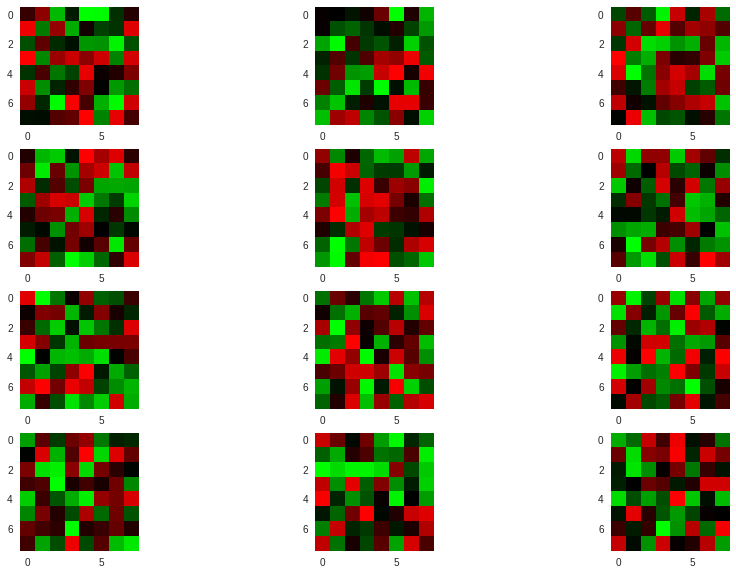## DCT Domain Batch Normalization¶

Batch normalization in the DCTdomain is also quite simple and includes some interesting performance enhancements. Our standard DCT implementation is copied below with a helper function to apply the DCT to each channel of the batch of images.

In :
def normalize(N):
n = np.ones((N, 1))
n[0, 0] = 1 / np.sqrt(2)
return (n @ n.T)

def harmonics(N):
spatial = np.arange(N).reshape((N, 1))
spectral = np.arange(N).reshape((1, N))

spatial = 2 * spatial + 1
spectral = (spectral * np.pi) / (2 * N)

return np.cos(spatial @ spectral)

def dct(im):
N = im.shape

n = normalize(N)
h = harmonics(N)

coeff = (1 / np.sqrt(2 * N)) * n * (h.T @ im @ h)

return coeff

def idct(coeff):
N = coeff.shape

n = normalize(N)
h = harmonics(N)

im = (1 / np.sqrt(2 * N)) * (h @ (n * coeff) @ h.T)

return im

def batch_dct(batch):
return np.stack([np.stack([dct(batch[i, c, :, :]) for c in range(batch.shape)], axis=0) for i in range(batch.shape)], axis=0)

def batch_idct(batch):
return np.stack([np.stack([idct(batch[i, c, :, :]) for c in range(batch.shape)], axis=0) for i in range(batch.shape)], axis=0)


Next we develop the DCT batch normalization algorithm.

Recall that the $(i, j)$th DCT coefficient of an image $I$ is defined as

$$D_{i, j} = \frac{1}{\sqrt{2N}}C(i)C(j)\sum_x\sum_y I_{x, y}\cos\left(\frac{(2x+1)i\pi}{2N}\right)\cos\left(\frac{(2y+1)j\pi}{2N}\right)$$

And that $C(k) = \frac{1}{\sqrt{2}}$ for $k=0$, and 1 otherwise.

Then, for an $8 \times 8$ images, the $(0, 0)$ DCT coefficient is

$$D_{0, 0} = \frac{1}{\sqrt{2N}}\frac{1}{\sqrt{2}}\frac{1}{\sqrt{2}}\sum_x\sum_y I_{x, y}\cos\left(\frac{(2x+1)0\pi}{2N}\right)\cos\left(\frac{(2y+1)0\pi}{2N}\right) \\ = \frac{1}{\sqrt{2N}}\frac{1}{\sqrt{2}}\frac{1}{\sqrt{2}}\sum_x\sum_y I_{x, y}\cos(0)\cos(0) \\ = \frac{1}{\sqrt{2N}}\frac{1}{\sqrt{2}}\frac{1}{\sqrt{2}}\sum_x\sum_y I_{x, y} \\ = \frac{1}{\sqrt{2 \cdot 8}}\frac{1}{\sqrt{2}}\frac{1}{\sqrt{2}}\sum_x\sum_y I_{x, y} \\ = \frac{1}{\sqrt{16}}\frac{1}{2}\sum_x\sum_y I_{x, y} \\ = \frac{1}{4}\frac{1}{2}\sum_x\sum_y I_{x, y} \\ = \frac{1}{8}\sum_x\sum_y I_{x, y} \\$$

Which is exactly the 8 times the mean of the block (there are 64 samples in a $8 \times 8$ block). This means that in order to zero-mean the image, we need only set the $(0,0)$ coefficient to zero without doing anything else. Compare this to computing the mean in the spatial domain, which requires $w \times h$ adds and a multiply, then subtracting it from each pixel which requires another $w \times h$ adds. To do this for the entire batch of DCT transformed images, then, simply requires $n \times c$ unconditional sets, rather than the $2 \times x \times w \times h \times c$ total adds and 1 multiply. This is a massive increase in efficiency for the same result.

Next we must scale by the std deviation. For this we can use the following theorem to help:

DCT Mean Variance Theorem Let $x = [x_0, ..., x_n]$ be a vector of samples such that $\mathrm{E}[x] = 0$. Let $y = [y_0, ..., y_n]$ be the DCT coefficients of $x$. Then $$\mathrm{Var}[x] = \mathrm{E}[y^2]$$

This is easy to show given that the DCT is an orthonormal transform. Proof is given in the appendix to these notes.

Also note that because of the linearity of the DCT, multiplying each pixel in the original image by the same constant is equivilent to multiplying the DCT coefficients by the same constant (this was covered in the "Understanding the DCT" notebook).

These two results imply a simple algorithm for scaling the DCT transformed images. Simply square the DCT coefficients, compute their mean, then divide the DCT coefficients by that result. Note that this is no worse than the scaling operation in the spatial domain. However, because the DCT of an image, especially if it was a result of JPEG decompression, is extremely sparse. This means that a sparse aware datastructure can simply noop for many steps of the computation, something that is impossible for most spatial domain images.

The full algorithm is implemented below on the DCT of the original batch. Note that it gives exactly the result of the spatial domain algorithm to within a floating point error. For reference, the means and std devations are printed for comparison with the spatial domain algorithm. Note that because our theorem defines the variance for each block, it cannot be used to directly compute the variance of several blocks over the batch. This is why the blockwise variances must be computed separately and merged to produce the population variance.

In :
dct_batch = batch_dct(batch)

def dct_norm(batch):
normed = batch.copy()
batch_channels = normed.transpose((1, 0, 2, 3))

m = np.mean(batch_channels[:, :, 0, 0], axis=1) / 8

m_blocks = batch_channels[:, :, 0, 0] / 8
batch_channels[:, :, 0, 0] = 0
v_blocks = np.mean(batch_channels**2, axis=(2, 3))

var_total = np.sum(v_blocks + m_blocks**2, axis=1) / batch.shape - m**2
s = np.sqrt(var_total)

normed /= s.reshape(1, -1, 1, 1)

print('Original Mean: {}, StdDev: {}'.format(m, s))

return normed

spectral_normed = dct_norm(dct_batch)

show_batch(batch_idct(spectral_normed))

print('New Mean: {}, StdDev: {}'.format(np.mean(batch_idct(spectral_normed), axis=(0, 2, 3)), np.std(batch_idct(spectral_normed), axis=(0, 2, 3))))

Original Mean: [126.171875   129.15234375 131.3203125 ], StdDev: [71.25412372 72.17629942 71.8572732 ]
New Mean: [5.11743425e-17 0.00000000e+00 5.20417043e-18], StdDev: [0.99796261 0.99126314 0.99717673]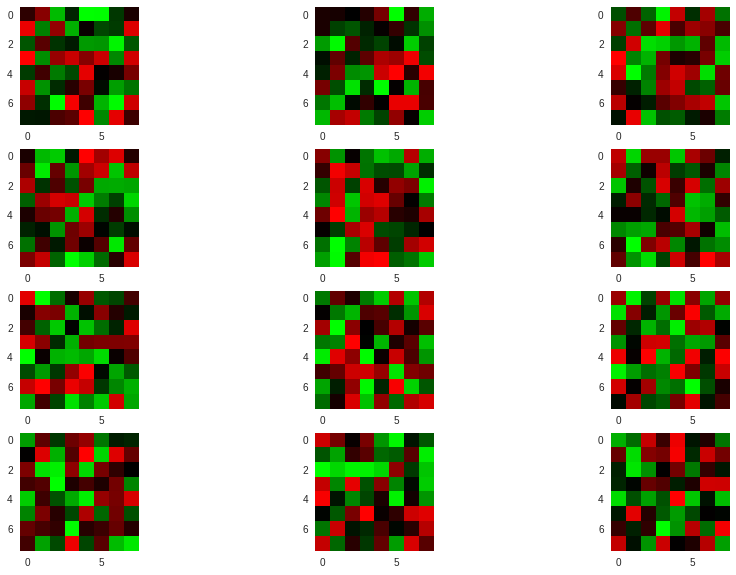## What About $\gamma$ and $\beta$?¶

Application of $\gamma$ and $\beta$ are quite simple in the spatial domain. Their application is also simple in the DCT domain, but it deserves discussion. Below, a $\gamma$ and $\beta$ are picked at random.

In :
gamma = np.random.randint(0, 255, size=batch.shape)
beta = np.random.randint(0, 255, size=batch.shape)

print('Beta: {}, Gamma: {}'.format(beta, gamma))

Beta: [ 92 110  74], Gamma: [  4  13 157]


Next they are applied to the spatial domain normalized batch. Note that the new mean is equal to $\beta$ and the new std deviation is equal to $\gamma$.

In :
spatial_bn = spatial_normed * gamma.reshape((1, -1, 1, 1)) + beta.reshape(1, -1, 1, 1)
show_batch(spatial_bn)

print('New Mean: {}, StdDev: {}'.format(np.mean(spatial_bn, axis=(0, 2, 3)), np.std(spatial_bn, axis=(0, 2, 3))))

New Mean: [ 92. 110.  74.], StdDev: [  4.  13. 157.]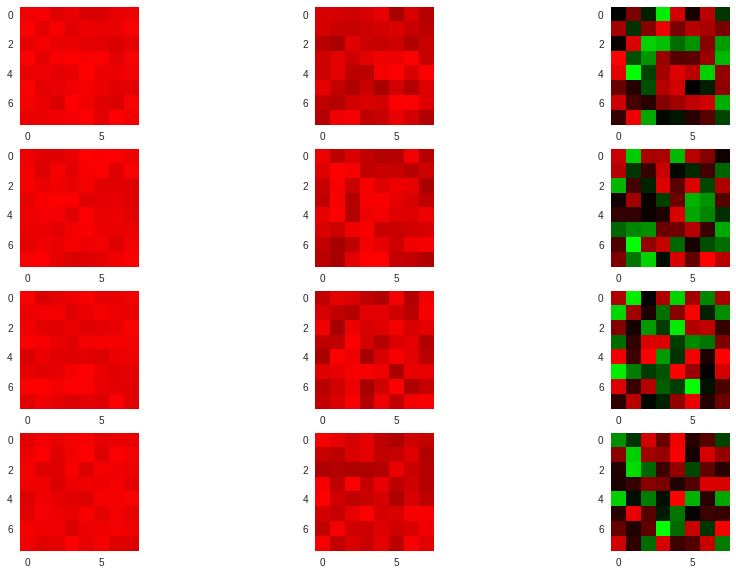Next the same $\gamma$ and $\beta$ are applied to the DCT domain normalized images. As in the discussion of the normalization above, applying $\gamma$ is as simple as multiplying the DCT coefficients as in the spatial domain. Applying $\beta$ however is much easier with a similar optimization as zeroing the mean. Instead we simply add to the $(0, 0)$th DCT coefficient $8\beta$. Since the image was previous normalized, this can be further optmized to an unconditional set. Thats one multiply and one unconditional set per image in the batch vs $n \times w \times h$ adds.

In :
spectral_bn = spectral_normed.copy()
spectral_bn = spectral_bn * gamma.reshape((1, -1, 1, 1))
spectral_bn[:, :, 0, 0] = beta * 8

show_batch(batch_idct(spectral_bn))

print('New Mean: {}, StdDev: {}'.format(np.mean(batch_idct(spectral_bn), axis=(0, 2, 3)), np.std(batch_idct(spectral_bn), axis=(0, 2, 3))))

New Mean: [ 92. 110.  74.], StdDev: [  3.99185042  12.88642078 156.55674671]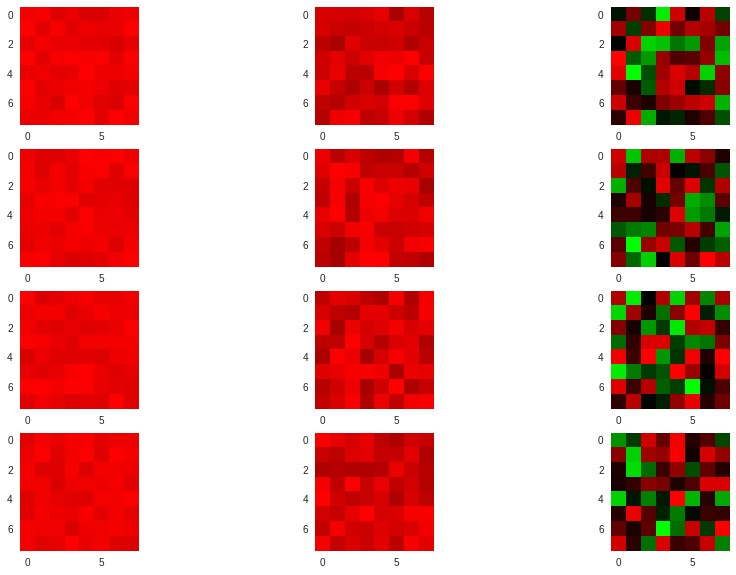Remember that at inference time, the parameters $\beta$ and $\gamma$ are replaced by

$$\gamma' = \frac{\gamma}{\sqrt{\mathrm{Var}[x]}} \\ \beta' = \beta - \frac{\gamma\mathrm{E}[x]}{\sqrt{\mathrm{Var}[x]}}$$

which can be applied using the same technique described above.

© 2018 Max Ehrlich